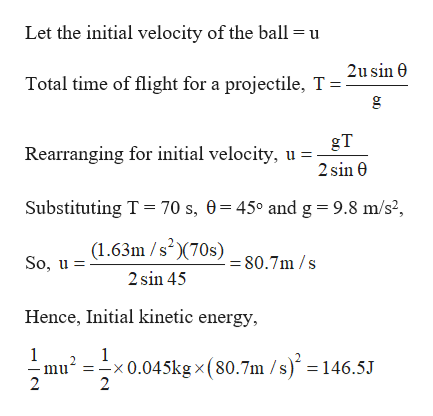# Apollo 14 astronaut Alan Shepard famously took two golfshots on the Moon where it’s been estimated that an expertlyhit shot could travel for 70.0 s through the Moon’s reducedgravity, airless environment to a maximum range of 4.00 km(about 2.5 miles). Assuming such an expert shot has a launchangle of 45.0°, determine the golf ball’s (a) kinetic energy asit leaves the club, and (b) maximum altitude in km above thelunar surface. Take the mass of a golf ball to be 0.045 0 kg andthe Moon’s gravitational acceleration to be gmoon = 1.63 m/s2.

Question
1 views

Apollo 14 astronaut Alan Shepard famously took two golf
shots on the Moon where it’s been estimated that an expertly
hit shot could travel for 70.0 s through the Moon’s reduced
gravity, airless environment to a maximum range of 4.00 km
(about 2.5 miles). Assuming such an expert shot has a launch
angle of 45.0°, determine the golf ball’s (a) kinetic energy as
it leaves the club, and (b) maximum altitude in km above the
lunar surface. Take the mass of a golf ball to be 0.045 0 kg and
the Moon’s gravitational acceleration to be gmoon = 1.63 m/s2.

check_circle

Step 1

Given:

Mass of the projectile ball, m = 0.045 kg

Range = 4 km = 4000 m

Angle of projection = 45 degrees

Time of flight = 70 s

Moon’s gravitational acceleration to be gmoon = 1.63 m/s2

Step 2

Calculating the kinetic energy of the ball as it leaves the surface:

...help_outlineImage TranscriptioncloseLet the initial velocity of the ball = u 2u sin 0 Total time of flight for a projectile, T= gT Rearranging for initial velocity, u = 2 sin 0 Substituting T = 70 s, 0=45° and g=9.8 m/s², (1.63m /s)(70s) So, u = = 80.7m /s 2 sin 45 Hence, Initial kinetic energy, -x0.045kg × (80.7m /s) = 146.5J fullscreen

### Want to see the full answer?

See Solution

#### Want to see this answer and more?

Solutions are written by subject experts who are available 24/7. Questions are typically answered within 1 hour.*

See Solution
*Response times may vary by subject and question.
Tagged in

### Kinematics